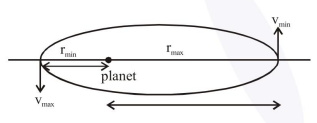# A satellite is in an elliptical orbit around a planet P.

Question:

A satellite is in an elliptical orbit around a planet P. It is observed that the velocity of the satellite when it is farthest from the planet is 6 times less than that when it is closest to the planet. The ratio of distances between the satellite and the planet at closest and farthest points is :

1. $1: 6$

2. $3: 4$

3. $1: 3$

4. $1: 2$

Correct Option: 1,

Solution:By angular momentum conservation

$\mathrm{r}_{\min } \mathrm{v}_{\max }=\mathrm{r}_{\max } \mathrm{v}_{\min }$.......(1)

Given $\mathrm{V}_{\min }=\frac{\mathrm{v}_{\max }}{6}$

from equation (i)

$\frac{r_{\min }}{r_{\max }}=\frac{v_{\min }}{v_{\max }}=\frac{1}{6}$

Ans. (1)